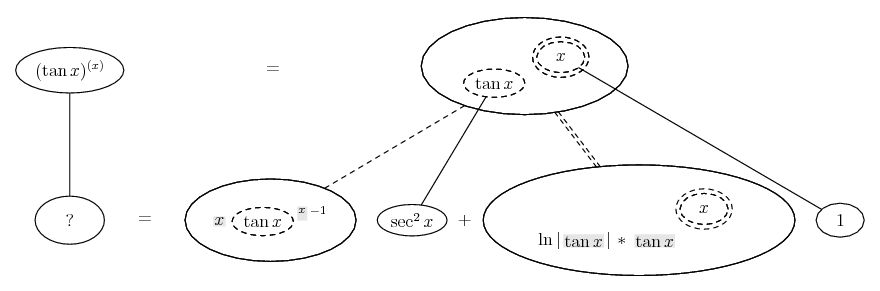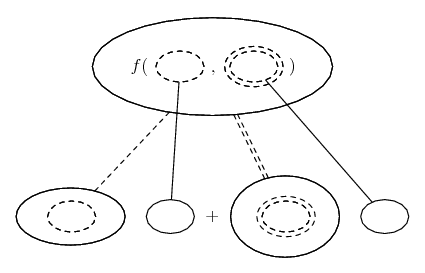# Thread: Derivative of (tan(x))^x ?? :s

1. ## Derivative of (tan(x))^x ?? :s

been trying to this for some time now dont get it lol :S

$\displaystyle (tan(x))^x$

i tried chain rule :s

let y=u^x and u=tan(x)

y'=ln(u)/u , u'=sec^2(x)

$\displaystyle \frac{dy}{dx}=\frac{sec^2(x).ln|tan(x)|}{tan(x)}$

but ans is $\displaystyle [ln|tan(x)|+2xcosec(2x)](tan(x))^x$

2. The standard method is to take natural logs of both sides and then differentiate...

However, - Wolfram|Alpha does it with the chain rule for two inner functions...

$\displaystyle \frac{d}{dx}\ f(u(x), v(x)) = \frac{\partial f}{\partial u} \frac{du}{dx} + \frac{\partial f}{\partial v} \frac{dv}{dx}$

... which doesn't mean we have to, of course, but I fancy this picture makes it palatable...... where... is the double version of...... the ordinary chain rule. As with that, straight continuous lines differentiate downwards (integrate up) with respect to x, and the straight dashed lines similarly but with respect to the (corresponding) dashed balloon expression which is (one of) the inner function(s) of the composite expression.

Shading shows that an expression has been treated as a constant on the way down (during differentiation).

You do, admittedly, need logarithmic differentiation to see the logic of the double-dashed differentiation, i.e. the logic of...

$\displaystyle \frac{d}{du}\ a^u = \ln a\ a^u$

_____________________________________

Don't integrate - balloontegrate!

Balloon Calculus: Standard Integrals, Derivatives and Methods

Balloon Calculus Drawing with LaTeX and Asymptote!

3. I kinda get the jist of what you posted but can you clarify what the lines are getting confused by your solid, dashed, dobule dashed lines lol :S

4. Sure - travelling down a dashed line means differentiating with respect to the dashed balloon, so that other expressions are held as constants. So it's all just equivalent to the two-variable chain rule - which I'll fill out in a minute. Meanwhile, why not click the Wolfram link and click there on 'show steps'...

$\displaystyle \frac{d}{dx}\ f(u(x), v(x)) = \frac{\partial f}{\partial u} \frac{du}{dx} + \frac{\partial f}{\partial v} \frac{dv}{dx}$

So...

$\displaystyle \frac{d}{dx}\ (\tan x)^x = \frac{\partial}{\partial (\tan x)}\ (\tan x)^x \frac{d}{dx}\ \tan x + \frac{\partial}{\partial x}\ (\tan x)^x \frac{d}{dx}\ x$

Notation here possibly not entirely kosher, but I hope it helps.

5. Alright cool thanks i understood your way and i also tried the differentiation by logs method and both gave me the following answer.

$\displaystyle \frac{dy}{dx}=(tan(x))^x[(x(sec(x)csc(x)))+ln|tan(x)|]$

but i don't see how in the back of the book they got it as this form

$\displaystyle [ln|tan(x)|+2xcosec(2x)](tan(x))^x$

someone explain somehow they simplified

$\displaystyle (x(sec(x)csc(x)))$ to $\displaystyle 2xcsc(2x)$

thankkks

6. sin(2x) = 2 sin x cos x

7. mmmm not sure :X

trying this

csc(x)sec(x)=1/sin(x)cos(x)

using 1/2sin(2x)=sin(x)cos(x)

csc(x)sec(x)=1/[1/2sin2x] = 2sin2x = 2[2cosxsinx] but i dont c me getting newhere near 2csc2x from there on

8.Originally Posted by Kevlarmmmm not sure :X

trying this

csc(x)sec(x)=1/(sin(x)cos(x))

using 1/2sin(2x)=sin(x)cos(x)

csc(x)sec(x)=1/[(1/2)sin2x] = 2/sin(2x) ...
Nearly there

#### Search Tags

derivative, tanxx